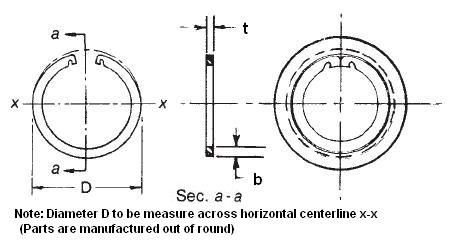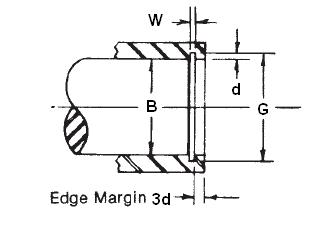### IN-200

Display Units: inches |  metric
Request Quick Quote for this part.
 Ring Specs (D) Free Diameter: 2.147 +0.070 / -0.000 in. (t) Thickness: 0.062 +0.002 / -0.002 in. (b) Radial Wall: 0.156 +0.005 / -0.005 in. Groove Specs: (B) Application Diameter: 2.000 in. (G) Groove Diameter: 2.122 +0.005 / -0.005 in. (W) Groove Width: 0.068 +0.004 / -0.000 in. Groove Depth: 0.061 in. Other Specs Approximate Weight per 1000: (GAPmin) Gap Minimum: 0.420 in. (ND) Notch Depth (+.000 / -.030 "): 0.078 in. (NW) Notch Width (Ref.): 0.093 in. (Pg) Theoretical Thrust Load Capacity - Groove Yield:Notes: Yield Strength of Groove Material (Ys): 45,000 psi. Calculated using a safety factor (K) of 4Equation:Pg = [ B * d * Ys * pi ] / K    = [ (2.000 in.) * (0.061 in.) * (45,000 psi) * 3.14 ] / 4 4,309 lbs. (Pr) Theoretical Thrust Load Capacity - Ring Shear:Notes: Ring Material: Carbon Spring Steel (SAE 1060-1095). Shear Strength of Ring Material (Ss): 153,000 psi. Calculated using a safety factor (K) of 3Equation:Pr = [ B * t * Ss * pi ] / K    = [ (2.000 in.) * (0.062 in.) * (153,000 psi) * 3.14 ] / 3 19,857 lbs. Industry Equivalent Part Number(s):IN-200